# 1.12: Drawing Chemical Structures

$$\newcommand{\vecs}{\overset { \rightharpoonup} {\mathbf{#1}} }$$ $$\newcommand{\vecd}{\overset{-\!-\!\rightharpoonup}{\vphantom{a}\smash {#1}}}$$$$\newcommand{\id}{\mathrm{id}}$$ $$\newcommand{\Span}{\mathrm{span}}$$ $$\newcommand{\kernel}{\mathrm{null}\,}$$ $$\newcommand{\range}{\mathrm{range}\,}$$ $$\newcommand{\RealPart}{\mathrm{Re}}$$ $$\newcommand{\ImaginaryPart}{\mathrm{Im}}$$ $$\newcommand{\Argument}{\mathrm{Arg}}$$ $$\newcommand{\norm}{\| #1 \|}$$ $$\newcommand{\inner}{\langle #1, #2 \rangle}$$ $$\newcommand{\Span}{\mathrm{span}}$$ $$\newcommand{\id}{\mathrm{id}}$$ $$\newcommand{\Span}{\mathrm{span}}$$ $$\newcommand{\kernel}{\mathrm{null}\,}$$ $$\newcommand{\range}{\mathrm{range}\,}$$ $$\newcommand{\RealPart}{\mathrm{Re}}$$ $$\newcommand{\ImaginaryPart}{\mathrm{Im}}$$ $$\newcommand{\Argument}{\mathrm{Arg}}$$ $$\newcommand{\norm}{\| #1 \|}$$ $$\newcommand{\inner}{\langle #1, #2 \rangle}$$ $$\newcommand{\Span}{\mathrm{span}}$$$$\newcommand{\AA}{\unicode[.8,0]{x212B}}$$

##### Objectives

After completing this section, you should be able to

1. propose one or more acceptable Kekulé structures (structural formulas) for any given molecular formula
2. write the molecular formula of a compound, given its Kekulé structure.
3. draw the shorthand structure of a compound, given its Kekulé structure.
4. interpret shorthand structures and convert them to Kekulé structures.
5. write the molecular formula of a compound, given its shorthand structure.
##### Study Notes

When drawing the structure of a neutral organic compound, you will find it helpful to remember that

• each carbon atom has four bonds.
• each nitrogen atom has three bonds.
• each oxygen atom has two bonds.
• each hydrogen atom has one bond.

Through general chemistry, you may have already experienced looking at molecular structures using Lewis structures. Because organic chemistry can involve large molecules it would be beneficial if Lewis structures could be abbreviated. The three different ways to draw organic molecules include Keku Formulas, Condensed Formulas, and Skeletal structures (also called line-bond structures or line formulas). During this course, you will view molecules written in all three forms. It will be more helpful if you become comfortable going from one style of drawing to another, and look at drawings and understanding what they represent. Developing the ability to convert between different types of formulas requires practice, and in most cases the aid of molecular models. Many kinds of model kits are available to students and professional chemists, and the beginning student is encouraged to obtain one.

Simplification of structural formulas may be achieved without any loss of the information they convey. Kekule formulas is just organic chemistry's term for Lewis structures you have previously encountered. In condensed structural formulas, the bonds to each carbon are omitted, but each distinct structural unit (group) is written with subscript numbers designating multiple substituents, including the hydrogens. Line formulas omit the symbols for carbon and hydrogen entirely (unless the hydrogen is bonded to an atom other than carbon). Each straight line segment represents a bond, the ends and intersections of the lines are carbon atoms, and the correct number of hydrogens is calculated from the tetravalency of carbon. Non-bonding valence shell electrons are omitted in these formulas.

## Kekulé (a.k.a. Lewis Structures)

A Kekulé Formula or structural formula displays the atoms of the molecule in the order they are bonded. It also depicts how the atoms are bonded to one another, for example single, double, and triple covalent bond. Covalent bonds are shown using lines. The number of dashes indicate whether the bond is a single, double, or triple covalent bond. All atom labels are shown and all lone pairs are shown.A B C

### Condensed Formula

A condensed formula is made up of the elemental symbols. Condensed structural formulas show the order of atoms like a structural formula but are written in a single line to save space and make it more convenient and faster to write out. The order of the atoms suggests the connectivity in the molecule. Condensed structural formulas are also helpful when showing that a group of atoms is connected to a single atom in a compound. When this happens, parenthesis are used around the group of atoms to show they are together. Also, if more than one of the same substituent is attached to a given atom, it is show with a subscript number. An example is CH4, which represents four hydrogens attached to the same carbon. Condensed formulas can be read from either direction and H3C is the same as CH3, although the latter is more common.

Look at the examples below and match them with their identical molecule under the Kekulé structures and the line formulas.

 CH3CH2OH ClCH2CH2CH(OCH3)CH3 CH3NHCH2COOH A B C

Let's look closely at example B. As you go through a condensed formula, you want to focus on the carbons and other elements that aren't hydrogen. The hydrogen's are important, but are usually there to complete octets. Also, notice the -OCH3 is in written in parentheses which tell you that it not part of the main chain of carbons. As you read through a a condensed formula, if you reach an atom that doesn't have a complete octet by the time you reach the next hydrogen, then it's possible that there are double or triple bonds. In example C, the carbon is double bonded to oxygen and single bonded to another oxygen. Notice how COOH means C(=O)-O-H instead of CH3-C-O-O-H because carbon does not have a complete octet and oxygens.

### Line Formula

Because organic compounds can be complex at times, line-angle formulas are used to write carbon and hydrogen atoms more efficiently by replacing the letter "C" with lines. A carbon atom is present wherever a line intersects another line. Hydrogen atoms are omitted but are assumed to be present to complete each of carbon's four bonds. Hydrogens that are attached to elements other than carbon are shown. Atom labels for all other elements are shown. Lone pair electrons are usually omitted. They are assumed to be present to complete the octet of non-carbon atoms. Line formulas help show the structure and order of the atoms in a compound.A B C

These molecules correspond to the exact same molecules depicted for Kekulé structures and condensed formulas. Notice how the carbons are no longer drawn in and are replaced by the ends and bends of a lines. In addition, the hydrogens have been omitted, but could be easily drawn in (see practice problems). Although we do not usually draw in the H's that are bonded to carbon, we do draw them in if they are connected to other atoms besides carbon (example is the OH group above in example A) . This is done because it is not always clear if the non-carbon atom is surrounded by lone pairs or hydrogens. Also in example A, notice how the OH is drawn with a bond to the second carbon, but it does not mean that there is a third carbon at the end of that bond/ line.

Kekulé Formula Condensed Formula Line FormulaCH3(CH2)3OHCH3CH2CH(OH)CH3(CH3)2CHCH2OH(CH3)3COHCH3CH2OCH2CH3Table $$\PageIndex{1}$$: Structural Formulas for C4H10O isomers

## Example: Converting between Structural Formulas

It is helpful to convert compounds into different structural formulas (Kekule, Line, and Condensed) depending on the type of question that is asked. Standardized exams frequently include a high percentage of condensed formulas because it is easier and cheaper to type letters and numbers than to import figures. Initially, it can be difficult writing a Line structure directly from a condensed formula. First, write the Kekule structure from the condensed formula and then draw the Line structure from the Kekule.

a) The condensed formula for propanal is CH3CH2CHO. Draw the Kekule structure.

The Kekule structure for propanal is shown below. Remember that every carbon will have four bonds and oxygens octet is filled with lone pairs.The bond-line structure for propanal is shown below. First, remove hydrogens. The hydrogen attached to the aldehyde group remains because it is part of a functional group. The remove the "C" labels from the structure and keep the lines in place. Lastly, remove any lone pairs.All three structures represent the same compound, propanal.b) The following is the line structure of the molecule trimethyl amine.To convert it to a Kekule structure first identify the carbons in the molecule. The will be at the corners and ends of line without an atom label. Trimethyl amine has three carbons. Next, add hydrogens to the carbons until four bonds are present. Each carbon in trimethyl amine is singly bonded to nitrogen. This means each carbon will need three additional C-H bonds to create its octet. Lastly, add lone pairs to other elements to fill their octets. The nitrogen in trimethyl amine is bonded to three carbons. This means it will require one of lone pair electrons to complete its octet.## Exercises

1. How many carbons are in the following drawing? How many hydrogens?2. How many carbons are in the following drawing? How many hydrogens?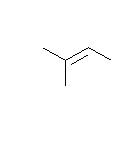3. How many carbons are in the following drawing? How many hydrogens?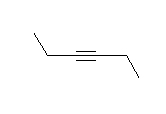4. Look at the following molecule of vitamin A and draw in the hidden hydrogens and electron pairs.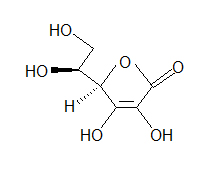(hint: Do all of the carbons have 4 bonds? Do all the oxygens have a full octet?)
5. Draw ClCH2CH2CH(OCH3)CH3 in Kekulé and line form.
6. Write down the molecular formula for each of the compounds shown here.1. Remember the octet rule and how many times carbons and hydrogens are able to bond to other atoms.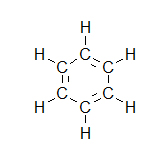2.3.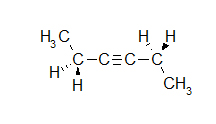4. Electron pairs drawn in blue and hydrogens draw in red.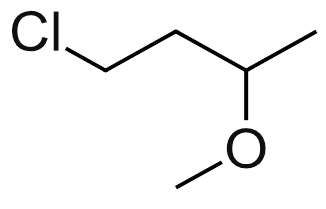6.

1. C7H7N
2. C5H10
3. C5H4O
4. C5H6Br2

#### Questions

Q1.12.1

Below is the molecule for caffeine. Give the molecular formula for it.#### Solutions

S1.12.1

C8H10O2N4

1.12: Drawing Chemical Structures is shared under a CC BY-SA 4.0 license and was authored, remixed, and/or curated by Steven Farmer, Dietmar Kennepohl, Krista Cunningham, Tim Soderberg, William Reusch, & William Reusch.